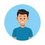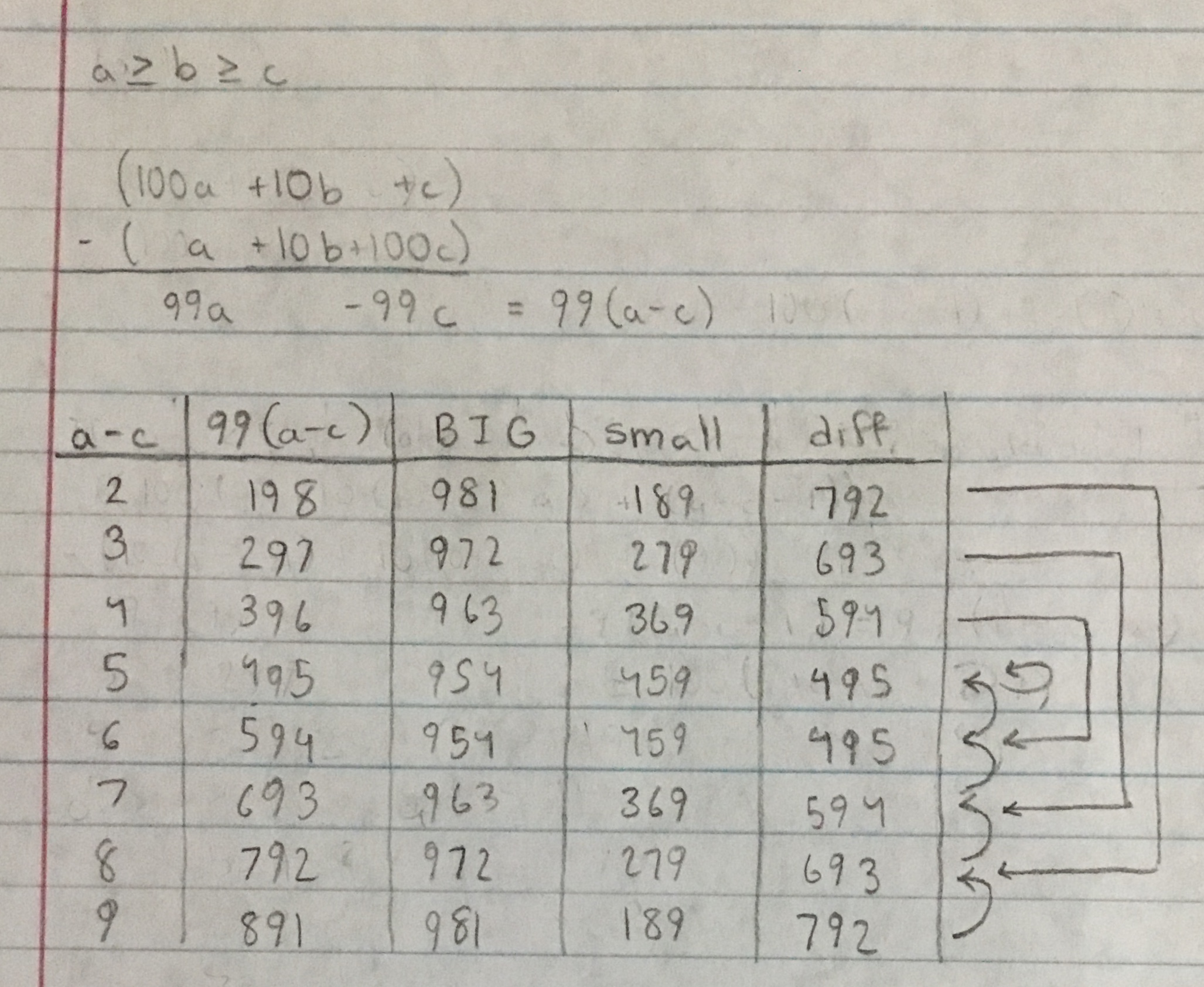# Can anyone share the proof for the following?

Today when I was surfing the internet I stumbled across this video.This was fasinating.

Take any 3 digit number, rearrange the numbers to form the largest number and the smallest number possible.

Subtract the two, repeat the process and eventually you will end at the number 495.

If you do this with any 4 digit number you get 6174.

I really wanted to know the proof of this. Can anybody please share the proof?PleaseNote by Shriniketan Ruppa
1 year, 1 month ago

This discussion board is a place to discuss our Daily Challenges and the math and science related to those challenges. Explanations are more than just a solution — they should explain the steps and thinking strategies that you used to obtain the solution. Comments should further the discussion of math and science.

When posting on Brilliant:

• Use the emojis to react to an explanation, whether you're congratulating a job well done , or just really confused .
• Ask specific questions about the challenge or the steps in somebody's explanation. Well-posed questions can add a lot to the discussion, but posting "I don't understand!" doesn't help anyone.
• Try to contribute something new to the discussion, whether it is an extension, generalization or other idea related to the challenge.

MarkdownAppears as
*italics* or _italics_ italics
**bold** or __bold__ bold
- bulleted- list
• bulleted
• list
1. numbered2. list
1. numbered
2. list
Note: you must add a full line of space before and after lists for them to show up correctly
paragraph 1paragraph 2

paragraph 1

paragraph 2

[example link](https://brilliant.org)example link
> This is a quote
This is a quote
    # I indented these lines
# 4 spaces, and now they show
# up as a code block.

print "hello world"
# I indented these lines
# 4 spaces, and now they show
# up as a code block.

print "hello world"
MathAppears as
Remember to wrap math in $$ ... $$ or $ ... $ to ensure proper formatting.
2 \times 3 $2 \times 3$
2^{34} $2^{34}$
a_{i-1} $a_{i-1}$
\frac{2}{3} $\frac{2}{3}$
\sqrt{2} $\sqrt{2}$
\sum_{i=1}^3 $\sum_{i=1}^3$
\sin \theta $\sin \theta$
\boxed{123} $\boxed{123}$

Sort by:

The algebraic proof is pretty intricate, and I'm too tired for $\LaTeX$, so here's a table of values.- 1 year, 1 month ago

If $a-c=0$ or $a-c=1$, the values leave 3 digit numbers and approach zero.

For four digit numbers, a similar proof can be used, but it might take more time.

- 1 year, 1 month ago

- 1 year, 1 month ago

I think it is a result by iteration.

- 1 year, 1 month ago

Some number which don't get to $495$:$111,222,333,444,555,666,777,888,999$

- 1 year, 1 month ago

@Zakir Husain : As you can see if you look in the reply to my comment, the numbers that don't converge to 495 are numbers where the difference between the biggest and smallest numbers are 1 or 0. For the numbers where this is 1 or 0, the sequence converges to 0.

- 1 year, 1 month ago